# Math in Focus Grade 3 Chapter 16 Practice 4 Answer Key Subtraction of Time

This handy Math in Focus Grade 3 Workbook Answer Key Chapter 16 Practice 4 Subtraction of Time provides detailed solutions for the textbook questions.

## Math in Focus Grade 3 Chapter 16 Practice 4 Answer Key Subtraction of Time

Subtract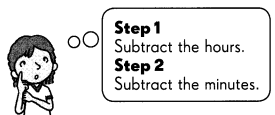Example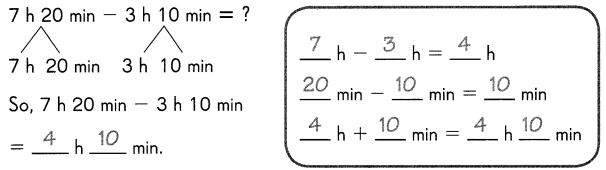Question 1.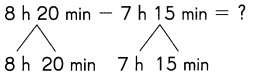So, 8 h 20 min – 7 h 15 min
= _______ h _________ min.

______ h – ______ h = ______ h
______ min – ______ min = ______ min
______ h – ______ min = ______ h ______ min
We measure time in hours, minutes, and seconds. There are 60 minutes in an hour and 60 seconds in a minute.
1. Subtract the hours
2. Subtract the minutes
3. If the minutes are negative, add 60 to the minutes and subtract 1 from hours.
Explanation:
Step 1: Subtract the hours.
=8hours-7hours
=1 hour
Step 2: Subtract the minutes.
=20min-15min
=5 min
Hence, the resultant from Step 1 and Step 2 together is the answer (1hour 5minutes).

Question 2.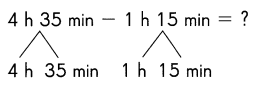So, 4 h 35 min – 1 h 15 min
= _______ h _________ min.

______ h – ______ h = ______ h
______ min – ______ min = ______ min
______ h – ______ min = ______ h ______ min
We measure time in hours, minutes, and seconds. There are 60 minutes in an hour and 60 seconds in a minute.
1. Subtract the hours
2. Subtract the minutes
3. If the minutes are negative, add 60 to the minutes and subtract 1 from hours.
Explanation:
Step 1: Subtract the hours.
=4hours-1hours
=3 hour
Step 2: Subtract the minutes.
=35min-15min
=20 min
Hence, the resultant from Step 1 and Step 2 together is the answer (3hour 20minutes).

Question 3.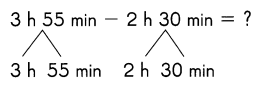So, 3 h 55 min – 2 h 30 min
= _______ h _________ min.

______ h – ______ h = ______ h
______ min – ______ min = ______ min
______ h – ______ min = ______ h ______ min
We measure time in hours, minutes, and seconds. There are 60 minutes in an hour and 60 seconds in a minute.
1. Subtract the hours
2. Subtract the minutes
3. If the minutes are negative, add 60 to the minutes and subtract 1 from hours.
Explanation:
Step 1: Subtract the hours.
=3hours-2hours
=1 hour
Step 2: Subtract the minutes.
=55min-30min
=25 min
Hence, the resultant from Step 1 and Step 2 together is the answer (1hour 25minutes).

Subtract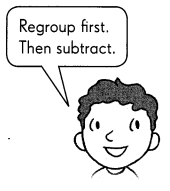Question 4.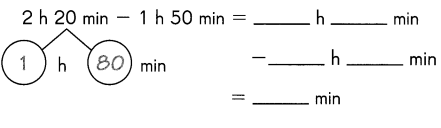Explanation:
We measure time in hours, minutes, and seconds. There are 60 minutes in an hour and 60 seconds in a minute.
1. Subtract the hours
2. Subtract the minutes
3. If the minutes are negative, add 60 to the minutes and subtract 1 from hours.
Here in the question, 2 h 20 min and 1 h 50 min.
Explanation:
Step 1: Subtract the hours.
=2hours-1hours
=1 hour
Step 2: Subtract the minutes.
=20min-50min
=-30 min
The minutes are negative. So follow step 3.
Step 3: add 60 to the minutes and subtract 1 from hours.
=60+20
=80
Subtract 1 from 2
=2-1
=1
Now calculate 1 h 80 min and 1 h 50 min
If we subtract hours 1-1=0
If we subtract min 80-50=30
Hence, the resultant from Step 1 and Step 2 together is the answer (0hour 30minutes).

Question 5.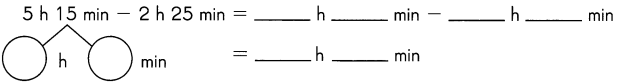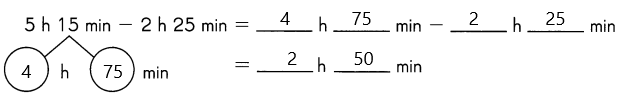Explanation:
1. Subtract the hours
2. Subtract the minutes
3. If the minutes are negative, add 60 to the minutes and subtract 1 from hours.
Here in the question, 2 h 20 min and 1 h 50 min.
Explanation:
Step 1: Subtract the hours.
=5hours-2hours
=3 hour
Step 2: Subtract the minutes.
=15min-25min
=-10 min
The minutes are negative. So follow step 3.
Step 3: add 60 to the minutes and subtract 1 from hours.
=60+15
=75
Subtract 1 from 2
=5-1
=4
Now calculate 4 h 75 min and 2 h 25 min
If we subtract hours 4-2=2
If we subtract min 75-25=50
Hence, the resultant from Step 1 and Step 2 together is the answer (2hour 50minutes).

Question 6.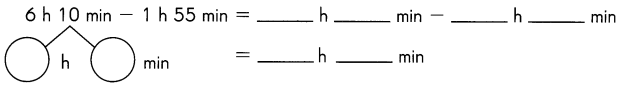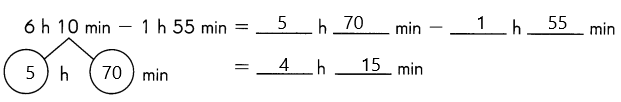Explanation:
1. Subtract the hours
2. Subtract the minutes
3. If the minutes are negative, add 60 to the minutes and subtract 1 from hours.
Here in the question, 6 h 10 min and 1 h 55 min.
Explanation:
Step 1: Subtract the hours.
=6hours-1hours
=5 hour
Step 2: Subtract the minutes.
=10min-55min
=-45 min
The minutes are negative. So follow step 3.
Step 3: add 60 to the minutes and subtract 1 from hours.
=10+60
=70
Subtract 1 from 6
=6-1
=5
Now calculate 5 h 70 min and 1 h 55 min
step 4: If we subtract hours 5-1=4
step 5: If we subtract min 70-55=15
Hence, the resultant from Step 4 and Step 5 together is the answer (4hour 15minutes).

Question 7.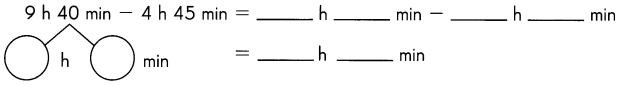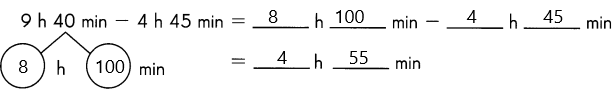Explanation:
1. Subtract the hours
2. Subtract the minutes
3. If the minutes are negative, add 60 to the minutes and subtract 1 from hours.
Here in the question, 9 h 40 min and 4 h 45 min.
Explanation:
Step 1: Subtract the hours.
=9hours-4hours
=5 hour
Step 2: Subtract the minutes.
=40min-45min
=-5 min
The minutes are negative. So follow step 3.
Step 3: add 60 to the minutes and subtract 1 from hours.
=40+60
=100
Subtract 1 from 9
=9-1
=8
Now calculate 8 h 100 min and 4 h 45 min
step 4: If we subtract hours 8-4=4
step 5: If we subtract min 100-45=55
Hence, the resultant from Step 4 and Step 5 together is the answer (4hour 55minutes).

Solve.

Question 8.
Rita takes 3 hours 5 minutes to sew a dress. Tara takes 2 hours 40 minutes to sew a similar dress. How much longer does Rita take to sew the dress than Tara?
Answer: 25 minutes longer Tita take to sew the dress than Tara.
The time Rita takes to sew a dress=3 h 5 minutes.
The time Tara takes to sew a dress=2 h 40 minutes.
1. Subtract the hours
2. Subtract the minutes
3. If the minutes are negative, add 60 to the minutes and subtract 1 from hours.
Here in the question, 3 h 5 min and 2 h 40 min.
Explanation:
Step 1: Subtract the hours.
=3hours-1hours
=1 hour
Step 2: Subtract the minutes.
=5min-40min
=-35 min
The minutes are negative. So follow step 3.
Step 3: add 60 to the minutes and subtract 1 from hours.
=60+5
=65
Subtract 1 from 3
=3-1
=2
Now calculate 2 h 65 min and 2 h 40 min
step 4: If we subtract hours 2-2=0
step 5: If we subtract min 65-40=25
Hence, the resultant from Step 4 and Step 5 together is the answer (0hour 25minutes).